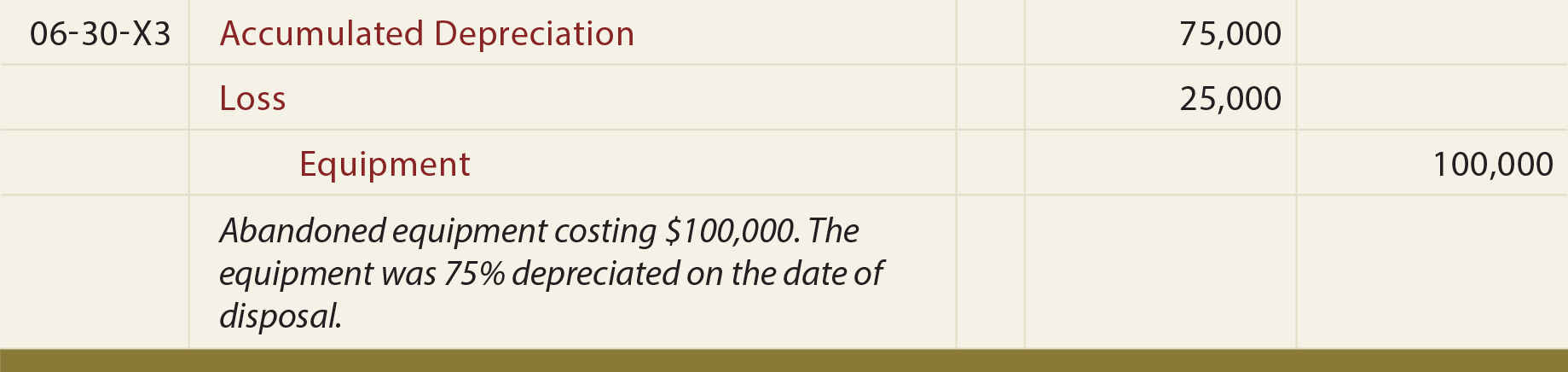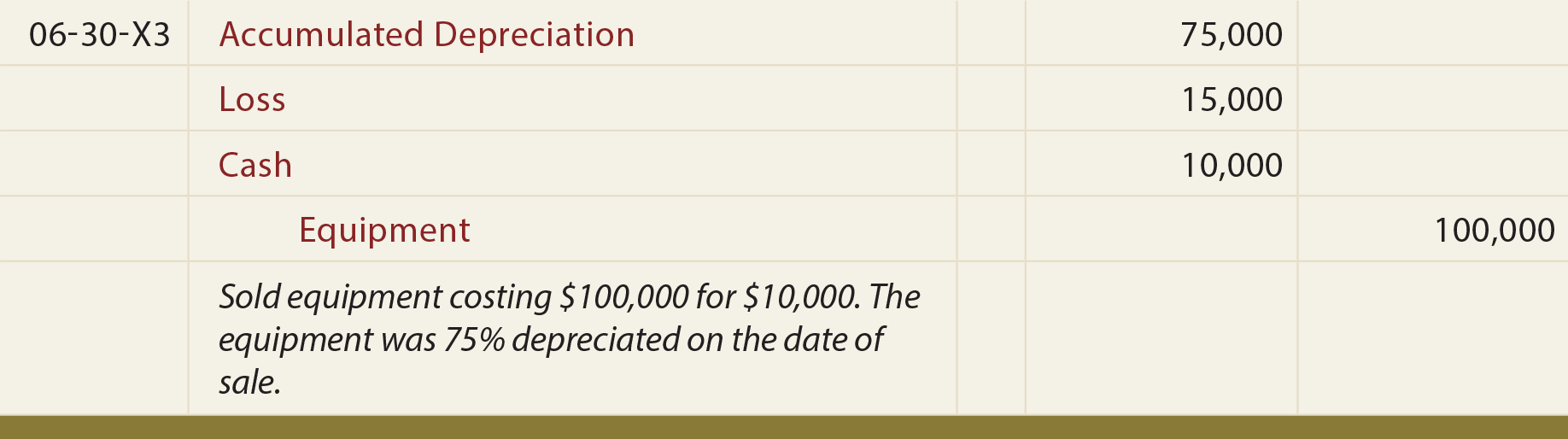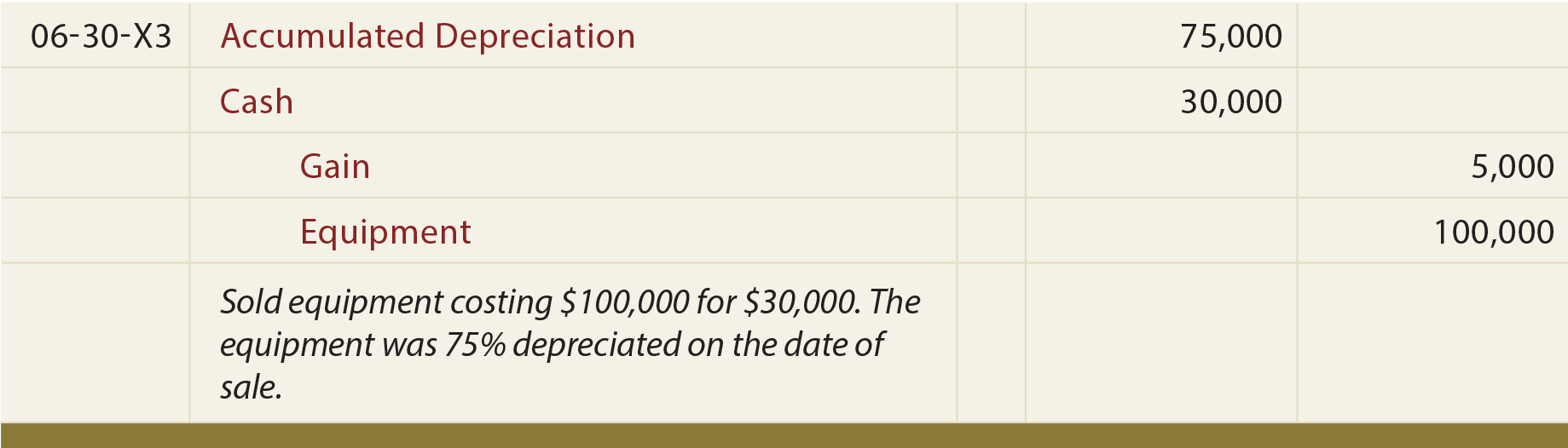Over time the productive assets in use by a company may no longer be needed and a decision is made to dispose of those assets. Disposal may occur by abandonment, sale, or exchange. In any case, it is necessary to update depreciation calculations through the date of disposal. Then, and only then, would the asset disposal be recorded.

If the asset is being scrapped (abandoned), the journal entry entails the elimination of the cost of the asset from the books, removal of the related accumulated depreciation, and potentially recording a loss to balance. This loss reflects the net book value that was not previously depreciated:On the other hand, an asset may be disposed of by sale, in which case the journal entry would need to be modified to include the proceeds of the sale. Assume the above asset was sold for \$10,000. The entry would be as follows:While the journal entry alone might be sufficient to demonstrate the loss calculation, one might also consider that an asset with a \$25,000 net book value is being sold for \$10,000. This gives rise to the loss of \$15,000.

Conversely, what if this asset were sold for \$30,000? In that case, the asset having a \$25,000 net book value is converted to \$30,000 cash. This triggers a \$5,000 gain. Simply stated a \$30,000 asset replaces an asset that was reported at \$25,000. Following is the entry for that scenario:Did you learn?
Be able to record the removal of a depreciable asset from the accounts.
Know how to record the sale of a depreciable asset, including situations involving either a gain or loss.